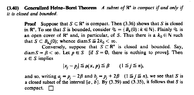# Heine-Borel Theorem in R^n .... Stromberg, Theorem 3.40 .... ....

• MHB
• Math Amateur

#### Math Amateur

Gold Member
MHB
I am reading Karl R. Stromberg's book: "An Introduction to Classical Real Analysis". ... ...

I am focused on Chapter 3: Limits and Continuity ... ...

I need help in order to fully understand the proof of Theorem 3.40 on page 104 ... ...

Theorem 3.40 and its proof read as follows:

View attachment 9141

In the above proof by Stromberg we read the following:

" ... ... To see that $$\displaystyle S$$ is bounded, consider $$\displaystyle \mathscr{U} = \{ B_k (0) \ : \ k \in \mathbb{N} \}$$. Plainly $$\displaystyle \mathscr{U}$$ is an open cover of $$\displaystyle \mathbb{R}^n$$ and, in particular of $$\displaystyle S$$. Thus there is a $$\displaystyle k_0 \in \mathbb{N}$$ such that $$\displaystyle S \subset B_{ k_0 } (0)$$; whence $$\displaystyle \text{diam}S \leq 2 k_0 \lt \infty$$. ... ... "

I am troubled by the apparent (to me) lack of a rigorous argument to demonstrate that $$\displaystyle S$$ is bounded ...

It appears to me that $$\displaystyle \mathbb{R}^n$$ is "infinitely large", that is unbounded ... and hence $$\displaystyle S$$ can be "infinitely large" and hence unbounded ... for example if $$\displaystyle S$$ is the $$\displaystyle x_1$$ axis of $$\displaystyle \mathbb{R}^n$$ then $$\displaystyle S \subset \mathbb{R}^n$$ and $$\displaystyle S$$ is unbounded ...

Can someone please demonstrate rigorously that $$\displaystyle S$$ is bounded ...

*** EDIT ***

Just a thought after reflection ... even though open covers of \mathbb{R}^n and S may be "infinite" (in dimension) because S is compact it must have a finite subcover ... and hence S is bounded ... is that the correct argument?

***************************************************************************************************

Help will be appreciated ...

Peter

#### Attachments

•Stromberg - Theorem 3.40 ... ... .png
18.9 KB · Views: 24
Last edited:

"Because S is compact it must have a finite subcover."

No, that is not correct. In fact, it doesn't make sense. To talk about a "subcover" you must first have specified a "cover". Are you talking about the one given in the argument, $B_k(0)$, the collection of open balls with center at 0 and radius k= 1, 2, 3, ...?

Notice that these balls are each contained in the next so that any point in the union of a finite number of them is in the largest, the largest "k". Since every point is S is some finite distance from 0 every point of S is in one of those so it is an open cover for S.

Since S is compact there exist a finite
subcover of that cover. There are only a finite number of such "$B_k(0)$" so there is a largest "k". S is covered by that single set so the distance between any two points in S is less than 2k.

"Because S is compact it must have a finite subcover."

No, that is not correct. In fact, it doesn't make sense. To talk about a "subcover" you must first have specified a "cover". Are you talking about the one given in the argument, $B_k(0)$, the collection of open balls with center at 0 and radius k= 1, 2, 3, ...?

Notice that these balls are each contained in the next so that any point in the union of a finite number of them is in the largest, the largest "k". Since every point is S is some finite distance from 0 every point of S is in one of those so it is an open cover for S.

Since S is compact there exist a finite
subcover of that cover. There are only a finite number of such "$B_k(0)$" so there is a largest "k". S is covered by that single set so the distance between any two points in S is less than 2k.

Thanks for the help, HallsofIvy ... ...

You write:

" ... ... No, that is not correct. In fact, it doesn't make sense. To talk about a "subcover" you must first have specified a "cover". Are you talking about the one given in the argument ... "

$$\displaystyle \mathscr{U} = \{ B_k (0) \ : \ k \in \mathbb{N} \}$$ as given by Stromberg ... but I did not say so explicitly ... so strictly speaking ... yes ... what I said was not correct ... indeed reading literally ... it didn't make sense ... as you say ...